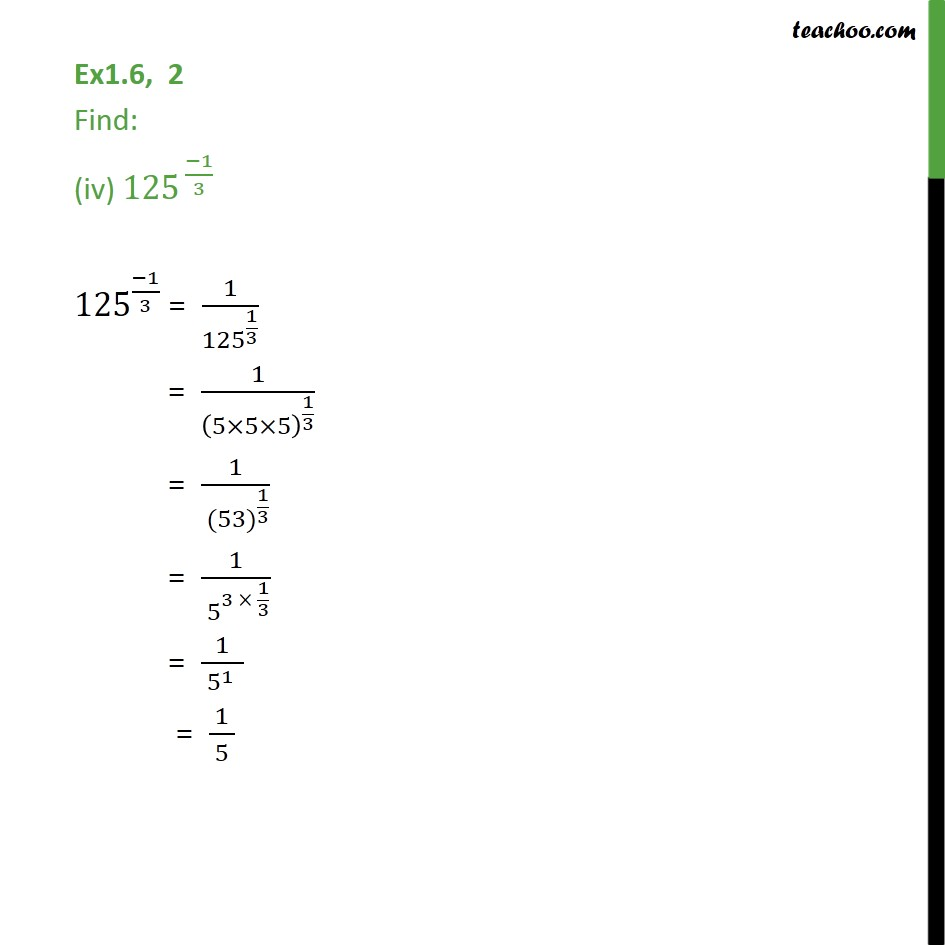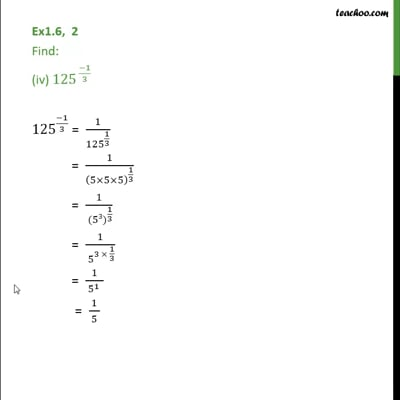Ex 1.6

Chapter 1 Class 9 Number Systems
Serial order wiseThis video is only available for Teachoo black users

Maths Crash Course - Live lectures + all videos + Real time Doubt solving!

### Transcript

Ex1.6, 2 Find: (iv) 〖125〗^( (−1)/3) 〖125〗^((−1)/3) = 1/〖125〗^(1/3) = 1/(5×5×5)^(1/3) = 1/〖 (53)〗^(1/3) = 1/〖 5〗^(3 × 1/3) = 1/〖 5〗^(1 ) = 1/〖 5〗^